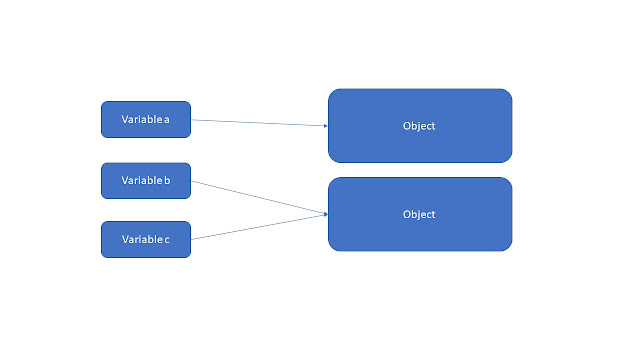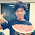[C#][Visual Studio] 資料型態與運算子

2017-08-13

## 介紹

#### 簡介

float b = 1.2;
char c = 'a';
bool e = true;
int f, g, i;參考資料：https://msdn.microsoft.com/en-us/library/ms228360(v=vs.90).aspx

1. class
2. interface
3. delegate
4. object
5. string#### 運算子

```int a = 10;
int b = 5;

int c = a + b;
int d = a - b;
int e = a * b;
int f = a / b;
int g = a % b;
```

++ 與 -- 為遞增與遞減，範例如下：

&& 與 || 為邏輯運算，比較常用於 if 判斷式 (下一篇流程控制會提到)，下表為邏輯判斷表：
AND 情況下，條件A與條件B必須都成立 (true)，否則皆為 false；OR 情況下，條件A與條件B必須都不成立 (false)，否則皆為 true。再說明範例前，我們先了解下列運算子。
```A == B // A 等於 B
A != B // A 不等於 B
A >= B // A 大於等於 B
A <= B // A 小於等於 B
```

#### 隱含轉換與明確轉換

```int a = 4;
long b = 5;
b = a;
```

```int a = (int) b;

string possibleInt = "1234";
int count = Convert.ToInt32(possibleInt);
```

#### 匿名類型

```var a = 10;
var op = new { age = 2};
```

#### 命名規範

```int backColor;
string currencyCode;
```

1. 顯露意圖：立刻解釋用途與如何使用
2. 不裝可愛與語帶雙關
3. 要念得出來
4. 盡可能使用專業術語
5. 一致性

## 參考資料

1. Study4TW 內部教材
2. 參考型別 - Microsoft Docs
3. Clean Code

#### { 2 意見... read them below or Comment }

1.chart c = 'a';
char多一個t

1.感謝提醒，已經修正，謝謝您 ^_^

- Copyright © Duran Hsieh @ Duran 的技術冶煉廠 - Date A Live - Powered by Blogger - Designed by Johanes Djogan -